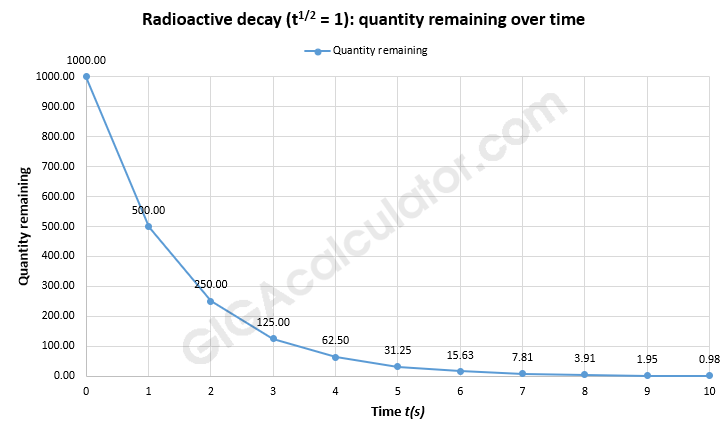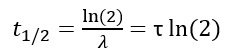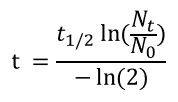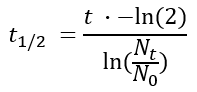# Half-Life Calculator

Use this decay calculator to easily calculate the time elapsed since the beginning of the decay, or calculate the original quantity, half-life (a.k.a. decay rate) or remaining quantity of a substance subject to radioactive decay, based on any of the three parameters. Convert half-life to mean lifetime or decay constant, and vice versa by entering any of the three values in its respective field.

### Calculation results

7,574.647983704585 y Time passed
Share calculator:

Embed this tool:
get code

## Using the Half-Life Calculator

Using the above multipurpose radioactive decay calculator you can:

• Time a sample if you know the current amount of radioactive matter in it, it's base (expected) amount and the half-life, decay constant or mean lifetime of the element you are measuring
• Calculate the half-life, decay constant and mean lifetime of an element if you have a sample for which you know the initial amount, the current amount, and the time passed between the two measurements.
• Calculate the initial quantity of a radioactive element, given its current quantity, its half-life (or decay constant, or mean lifetime) and the time it was decaying for.
• Calculate the remaining quantity of a radioactively decaying element given its initial quantity, its half-life (or decay constant, or mean lifetime), and the time it was decaying for.

Our versatile radioactive decay calculator supports many different time units and automatically converts them if the time unit you measure the time elapsed is different than the time unit you enter the half-time, decay constant or mean lifetime in. Supported units are nanoseconds, milliseconds, seconds, minutes, hours, days, weeks, months, and years. Months are approximated to 30.5 days, years are approximated to 365.2421 days. The half-life calculator results are accurate to the 16-th decimal and are usable in physics, chemistry, etc.

Radioactive decay (a.k.a. "nuclear decay", "radioactivity") is the process by which an unstable atomic nucleus loses energy (in terms of mass in its rest frame) by emitting radiation, such as an alpha particle, beta particle with neutrino or only a neutrino in the case of electron capture, gamma ray, or electron in the case of internal conversion. A material containing such unstable nuclei is considered radioactive. Certain highly excited short-lived nuclear states can decay through neutron emission, or more rarely, proton emission .

Since it is a stochastic (random) process, the decay rate of a particular atom cannot be predicted, but it can be for a group of atoms of the same element and this is the basis of radiometric dating. This particular quality also makes them a good source for random variation when generating true random numbers.

Different elements can have vastly different half lives. For example, on one end of the range we have carbon-8 with a half life of 2.0 x 10−21 s (0.000000000000002 nanoseconds), so this isotope can only be observed if produced artificially. On the other end of the spectrum we have Uranium-233 with a half-life of about 160 000 years.

## Half-Life, Decay Constant, and Mean Lifetime

While random at the individual level, radioactive decay is predictable over a group of particles with some uncertainty. It is an exponential process, meaning that the quantity of matter decreases at a rate proportional to its current value. The chart below shows an example in which the half of the particles decay each second and the plot reflects the particles remaining at seconds zero to ten:The most intuitive mathematical description of the rate of decay is half-life, which our half-life calculator can calculate. In the above graph the half-life time is exactly one second.

The relation between half-life, mean lifetime and the decay constant can be expressed by the equation:where t1/2 is the half-life of the particle, τ is the mean lifetime, λ is the decay constant, and ln is the natural logarithm. As you can see, conversion between these three is fairly trivial mathematically, and our decay calculator will handle it for you.

For example, knowing that the half-time of carbon-14 is about 5730 years (t1/2 = 5,730 years), and knowing that it is constantly produced in the atmosphere and consequently incorporated in plant matter during photosynthesis, you can measure the time since a living organism was alive and the current time fairly accurately for periods up to 50,000 years. This is what is commonly referred to as "carbon dating".

Let us say that you have a sample that you want to carbon date. You have estimated or measured that a living organism of that type and size is expected to contain 1,000 units of carbon-14 (N0 = 1,000). You have estimated that your sample presently contains about 400 units of carbon-14 (Nt = 400), or about 40% of the original amount. Plugging the numbers in the formula above, you will get that the organism was decaying for about 7,574 years, which result can easily be verified.

The formula for calculating the time elapsed from the beginning of the decay process to the current moment, or a chosen moment in the future, relative to the beginning of the decay is calculated using the formula:where t is the elapsed time, t1/2 is the half-life of the particle, N0 is the quantity in the beginning, and Nt is the quantity at time t. This is the equation used in our tool as well.

## Half-Life formula

You can find the half-life of a radioactive element using the formula:where t1/2 is the half-life of the particle, t is the elapsed time, N0 is the quantity in the beginning, and Nt is the quantity at time t. This equation is used in the calculator when solving for half-life time.

## Exponential decay applications

The applications of half-life calculation and exponential decay are many, as it has uses in electrostatics, chemical reaction rates, geophysics, archeology, fluid dynamics, heat transfer, optics, luminescence, pharmacology and toxicology, thermoelectricity, vibrations and, of course - radioactivity. There are even applications in finances and routing protocols in computer science.